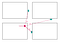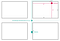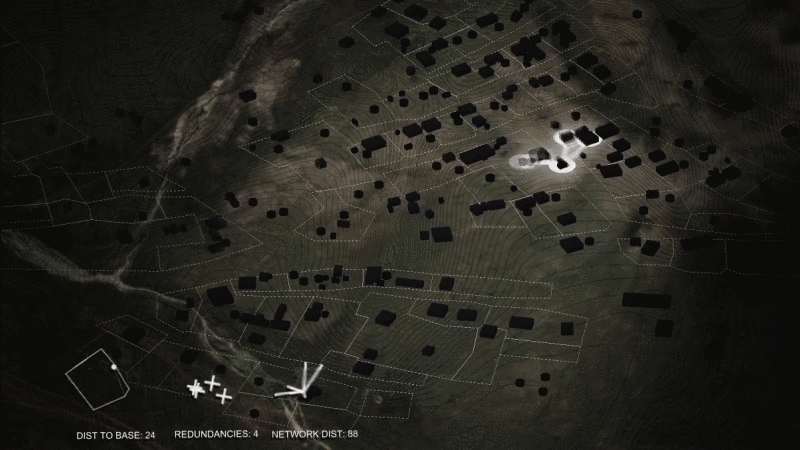# Uber Simulation Model

https://youtu.be/EQF7qo6OYnY

·Brief

This model provides a chance to evaluate the time people waiting for a taxi via application online which may be influenced by different elements.

To simplify this problem, the most important parameters that are taken into consideration are: 1. the frequency of cars coming from different directions; 2. the traffic congestion parameter(which is simplified into the velocity of cars in this model) 3. the frequency of customers who want a taxi. The grid of street is abstracted to several uniform blocks which are pretty typical in Manhattan.

·Major Algorithm

1. When generating a customer on the edge of each block, the first thing is to find the nearest uber in nearby area. Just access all the cars that have been generated and calculate the distance between the aim customer and each of them. Them by comparison the car agent that has accepted the order could be generated.D1 is the shortest so the bottom car will accept the customer.

2. Once the agent car has been confirmed, it should find the shortest way to accept the customer. This logic goes as follows:

1). Just keep going on the current direction until into the next cross, where it could turn direction.

2). On each crossroad, compare the coordinates of customer and the agent car. This information tells if the car should turn left/right or up/down.

3). What is the priority of turning direction? In order to go as short as possible, the agent car should go first on the direction which the relative distance is further.

4). There should be a value named P. Whenever the distance between the agent car and the customer is shorter than P, the agent car successfully accept the customer.The path in solid line is shorter than the path in dash line.

·Amendments

1. The cars should always go on the right side of roads. So there should be a judgement condition to tell if the car is not following traffic rule and turn to the right. This can be based on the relationship between the coordinates of car and current path and the vector of the car’s velocity.
2. There is chance that the agent car would not accept the customer. This happens in real life. So there should a trigger volume that if the agent car has gone away, this agent should be destroyed and the customer will find a new agent car. This kind of small accidents definitely make the waiting time longer.

·Harvest/Display

·Code Snippets

1. A customer calls a car

Including the algorithm of directing the agent car, drawing a line between agent car and customer, calculating time and the condition of accepting the customer.

`using System.Collections;using System.Collections.Generic;using UnityEngine;using UnityEditor;using System;using UnityEngine.UI;public class Customer : MonoBehaviour{float StartingTime=0;float EndTime=0;float DistancetoClosest=Mathf.Infinity;float DistancetoClosest2=Mathf.Infinity;float DistancetoClosest3=Mathf.Infinity;GameObject ClosestCar=null;GameObject LocalBlock=null;GameObject LocalCross=null;Vector3 UnitDirecting;float HorizontalUnits=20f;float VerticalUnits=10f;Rigidbody rb;void DestoryGameObj(){GameObject[] AllBlcoks=GameObject.FindGameObjectsWithTag("Block");foreach(GameObject Block in AllBlcoks){Vector3 VDistance=Block.transform.position-transform.position;VDistance.y=0f;float Distance=VDistance.sqrMagnitude;if(Distance<DistancetoClosest2){DistancetoClosest2=Distance;LocalBlock=Block;}}if((Math.Abs(transform.position.x-LocalBlock.transform.position.x)<9.5f&&Math.Abs(transform.position.z-LocalBlock.transform.position.z)<4.5f)||transform.position.y>1.5f||(Math.Abs(transform.position.x-LocalBlock.transform.position.x)>10f||Math.Abs(transform.position.z-LocalBlock.transform.position.z)>5f)){Destroy(this.gameObject);}}float timer;private void Turn(){GameObject[] AllCross= GameObject.FindGameObjectsWithTag("AAA");foreach(GameObject CurrentCross in AllCross){Vector3 VDistance=CurrentCross.transform.position-rb.position;VDistance.y=0f;float Distance=VDistance.sqrMagnitude;if(Distance<DistancetoClosest3)LocalCross=CurrentCross;if((LocalCross.transform.position-rb.position).sqrMagnitude<1f){if((rb.position.x-transform.position.x>3f)&&Mathf.Abs(rb.position.x-transform.position.x)/HorizontalUnits>=Mathf.Abs(rb.position.z-transform.position.z)/VerticalUnits){Debug.Log("A");Vector3 OldDirecting=UnitDirecting;UnitDirecting=new Vector3(-1f,0,0);if(Vector3.Scale(OldDirecting,UnitDirecting)==new Vector3(0,0,0)){ClosestCar.transform.Rotate(0f,90f,0f);}}else if((rb.position.x-transform.position.x<-3f)&&Mathf.Abs(rb.position.x-transform.position.x)/HorizontalUnits>=Mathf.Abs(rb.position.z-transform.position.z)/VerticalUnits){Debug.Log("B");Vector3 OldDirecting=UnitDirecting;UnitDirecting=new Vector3(1f,0,0);if(Vector3.Scale(OldDirecting,UnitDirecting)==new Vector3(0,0,0)){ClosestCar.transform.Rotate(0f,90f,0f);}}else if((rb.position.z-transform.position.z>3f)&&Mathf.Abs(rb.position.x-transform.position.x)/HorizontalUnits<Mathf.Abs(rb.position.z-transform.position.z)/VerticalUnits){Debug.Log("C");Vector3 OldDirecting=UnitDirecting;UnitDirecting=new Vector3(0,0,-1f);if(Vector3.Scale(OldDirecting,UnitDirecting)==new Vector3(0,0,0)){ClosestCar.transform.Rotate(0f,90f,0f);}} else if((rb.position.z-transform.position.z<-3f)&&Mathf.Abs(rb.position.x-transform.position.x)/HorizontalUnits<Mathf.Abs(rb.position.z-transform.position.z)/VerticalUnits){Debug.Log("D");Vector3 OldDirecting=UnitDirecting;UnitDirecting=new Vector3(0,0,1f);if(Vector3.Scale(OldDirecting,UnitDirecting)==new Vector3(0,0,0)){ClosestCar.transform.Rotate(0f,90f,0f);}}}}}void DirectingCar(){float constSpeed=4f;rb.velocity=constSpeed*UnitDirecting;if ((Math.Abs(rb.position.x-transform.position.x)<=3f&&Math.Abs(rb.position.z-transform.position.z)<=3f)||(rb.position-transform.position).sqrMagnitude<=11f){ClosestCar.tag="Serving";Counting.TradeCounter++;EndTime=Time.time;Counting.TotalTimer+=EndTime-StartingTime;UnitDirecting=rb.velocity.normalized;Destroy(this.gameObject);}}void UpandDown(){timer+=Time.deltaTime;if(timer>=0&&timer<1){GetComponent<Rigidbody>().velocity=new Vector3(0f,1f,0f);}if(timer>=1&&timer<2){GetComponent<Rigidbody>().velocity=new Vector3(0f,-1f,0f);timer-=2;}if (ClosestCar){Debug.DrawLine(ClosestCar.transform.position,transform.position);}}IEnumerator Waiting(){yield return new WaitForSeconds(5);}void FindCar(){GameObject[] AllCars= GameObject.FindGameObjectsWithTag("Cars");foreach(GameObject CurrentCar in AllCars){Vector3 VDistance=CurrentCar.transform.position-transform.position;VDistance.y=0f;float Distance=VDistance.sqrMagnitude;if(Distance<DistancetoClosest){DistancetoClosest=Distance;ClosestCar=CurrentCar;}}if(!ClosestCar)Destroy(this.gameObject);else{rb=ClosestCar.GetComponent<Rigidbody>();ClosestCar.tag="Called";UnitDirecting=ClosestCar.GetComponent<Rigidbody>().velocity.normalized;if((rb.position+UnitDirecting-this.transform.position).sqrMagnitude>(rb.position-this.transform.position).sqrMagnitude)UnitDirecting*=-1f;}}void Start(){DestoryGameObj();StartCoroutine(Waiting());FindCar();StartingTime=Time.time;}void Update(){if(!ClosestCar&&this.gameObject){FindCar();}if(rb){DirectingCar();Turn();DrawLine(this.gameObject.transform.position,rb.position+new Vector3(0,0.5f,0),Color.white);}if(!this.gameObject){if(ClosestCar)ClosestCar.tag="Cars";}UpandDown();}void DrawLine(Vector3 start, Vector3 end, Color color, float duration = 0.2f){GameObject myLine = new GameObject();myLine.transform.position = start;myLine.AddComponent<LineRenderer>();LineRenderer lr = myLine.GetComponent<LineRenderer>();lr.startColor=Color.Lerp(color,Color.magenta,0.8f);lr.endColor=Color.Lerp(color,Color.magenta,0.8f);lr.startWidth=0.005f;lr.endWidth=0.005f;lr.SetPosition(0, start);lr.SetPosition(1, end);GameObject.Destroy(myLine, duration);}}`

2. Car Generator

This is one of the four generator that randomly generating cars from four directions. When there is no customer, the cars will go straight and leave this area.

`using System.Collections;using System.Collections.Generic;using UnityEngine;public class Generator1 : MonoBehaviour{[SerializeField] float GeneratingPace;[SerializeField] float VelocityParameter;private float timer;public GameObject myCar;List<GameObject> newCar=new List<GameObject>();int i=0;Vector3 GeneratingPosition;void Start(){GeneratingPosition=GetComponent<Rigidbody>().position-new Vector3(Random.Range(-0.4f,0.4f),0f,0f);}void Update(){for(int k=0;k<=i-1;k++){if (newCar[k]&&newCar[k].tag=="Cars")newCar[k].GetComponent<Rigidbody>().velocity=new Vector3 (0f,0f,-1f) * VelocityParameter*Random.Range(0.7f,0.9f);}timer+=Time.deltaTime;if(timer>GeneratingPace*Random.Range(5f,50f)&&Random.Range(0,10)>8){i++;newCar.Add(Instantiate(myCar,GeneratingPosition,Quaternion.identity));GameObject p;GameObject q;p=newCar[i-1].transform.GetChild(0).gameObject;q=newCar[i-1].transform.GetChild(1).gameObject;p.GetComponent<Renderer>().material.color=Color.black;q.GetComponent<Renderer>().material.color=Color.black;timer-=GeneratingPace*1.5f;}}}`

3. People Generator

Each block has this script to generate customers.

`using System.Collections;using System.Collections.Generic;using UnityEngine;using UnityEngine.UI;public class PeopleGenerator : MonoBehaviour{[SerializeField] GameObject Customer;float timer;[SerializeField] float Frequency;void Start(){}void Update(){timer+=Time.deltaTime;if(timer>Frequency){int x=Random.Range(0,6);switch(x){case 0:{Instantiate(Customer,this.transform.position+new Vector3(9.5f,0.5f,Random.Range(-4.5f,4.5f)),Quaternion.identity);break;}case 1:{Instantiate(Customer,this.transform.position+new Vector3(-9.5f,0.5f,Random.Range(-4.5f,4.5f)),Quaternion.identity);break;}case 2:{Instantiate(Customer,this.transform.position+new Vector3(Random.Range(-9.5f,9.5f),0.5f,4.5f),Quaternion.identity);break;}case 3:{Instantiate(Customer,this.transform.position+new Vector3(Random.Range(-9.5f,9.5f),0.5f,-4.5f),Quaternion.identity);break;}default:{break;}}timer-=Frequency;}}}`

4. Drive On Right

The script attached on the prefab of roads which makes the car driving on them sticking to the right side.

`using System.Collections;using System.Collections.Generic;using UnityEngine;public class DriveOnRight : MonoBehaviour{private void OnTriggerStay(Collider other){if(other.gameObject.CompareTag("Cars")||other.gameObject.CompareTag("Called")||other.gameObject.CompareTag("Serving")){if(other.gameObject.transform.position.z>(this.gameObject.transform.position.z-0.3f)&&other.gameObject.GetComponent<Rigidbody>().velocity.x>0){other.gameObject.GetComponent<Rigidbody>().velocity+=new Vector3(0,0,-1f);}else if(other.gameObject.transform.position.z<(this.gameObject.transform.position.z+0.3f)&&other.gameObject.GetComponent<Rigidbody>().velocity.x<0){other.gameObject.GetComponent<Rigidbody>().velocity+=new Vector3(0,0,1f);}else if(other.gameObject.GetComponent<Rigidbody>().velocity.z!=0){other.gameObject.GetComponent<Rigidbody>().velocity=Vector3.Scale(other.gameObject.GetComponent<Rigidbody>().velocity,new Vector3(1,1,0));}}}}`

5. Counter

This is the script linked with UI text, counting the total trade numbers and calculating the average time.

`using System.Collections;using System.Collections.Generic;using UnityEngine;using UnityEngine.UI;public class Counting : MonoBehaviour{[SerializeField] public Text TradeCount, AverageTime;static public int TradeCounter;static public float TotalTimer;void Start(){TradeCounter=0;TotalTimer=0;updateText();}void Update(){updateText();}void updateText(){TradeCount.text="TradeCount: "+ TradeCounter.ToString();if(TradeCounter>0)AverageTime.text="AverageTime: "+ (TotalTimer/TradeCounter).ToString("F1")+"s";else{AverageTime.text="AverageTime: "+ "0.0s";}}}`

## Data Mining the City

#### Graduate course at Columbia University GSAPP, taught by Violet Whitney and TA Zeid Ghawi